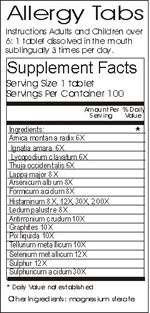Logic puzzles, riddles, math puzzles and brainteasers - pzzls.com

Vandaag is het 23 April 2021

# Quack medicine? - science puzzle

Difficulty:Rating: 2.8/5.0

Share this pzzl:

 According to homeopaths the effect of a homeopathic medicin is indicated by a dilution factor. Their theory is that if a substance is diluted more, the effect of it will increase. The dilution factor of a homeopathic medicin consists of a number and a character. An X or D indicates that the substance is diluted a number times 10. A C stands for a factor 100. As an example, 6 X indicates that the substance is diluted 10^6 = 1,000,000 times. Typical dilution factors of homeopathic medicins lie between 6 X and 30 X. Suppose you bought a litre of homepathic medicin with a dilution factor of 30 X. Assume that water was used for the dilution proces, and that the molecules of the substance are about as big as the water molecules (smaller is almost impossible, since water is one of the smallest molecules). How many molecules of the substance can you find in your bottle. And how many litres would you need to buy to be sure that you will have at least one molecule of the substance?# Explanation

Firstly, we should calculate the number of water molecules in a litre = 1000 g. For that we need the number of Avogadro, which says that in 1 mol substance consists of 6.02 * 10^23 molecules.

A mol of water (H20) weights (16 + 1 + 1) = 18 grams, which implies that 6.02 * 10^23 molecules of water weights 18 grams. A litre (1000 gram) of water hence consists of 6,02 * 10^23 / 0,018 = 3,34 * 10^25 molecules.

If the molecule of the substance is about as large as the water molecule, a dilution factor of 30 X implies that for every molecule of the substance, there should be 10^30 molecules of water. That's many more than you can find in a litre of water! Probably you won't find even a single molecule of the substance in the bottle.
If you need to be sure that you will have at least one molecule of the substance, you'll need 10^30 molecules of water. That is equal to 18*10^30 / (6,02 * 10^23) = 3,0 * 10^7 gram = 30.000 litres!

So dilution factors above 25 X are certainly quack medicine!